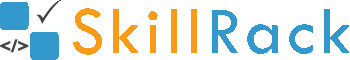Must Solve 100 Programs for Campus Placement Part - 10/104 months ago

1) Sum of Even Digits

The program must accept an integer N as the input. The program must print the sum of even digits in the integer N as the output.

Example Input/Output 1:
Input:
1427

Output:
6

Example Input/Output 2:
Input:
519

Output:
0

Click the link below to solve this program
http://www.skillrack.com/solve/9247

2) Maximum Length - S1 and S2

The program must accept two string values S1 and S2 as the input. The program must print the string having the maximum length as the output. If both the string values having the same length then print both the string values in the given order as the output.

Boundary Condition(s):
1 <= Length of S1, S2 <= 100

Example Input/Output 1:
Input:
rack
skill

Output:
skill

Click the link below to solve this program
http://www.skillrack.com/solve/9248

3) Print Integers - Right to X

The program must accept N unique integers and an integer X as the input. The program must print all the integers right to the integer X (inclusive of X) as the output.
Note: The integer X is always present in the N integers.

Boundary Condition(s):
2 <= N <= 100
1 <= Each integer value, X <= 1000

Example Input/Output 1:
Input:
6
20 35 10 44 99 18
44

Output:
44 99 18

Click the link below to solve this program
http://www.skillrack.com/solve/9249

4) Print Last K Integers

The program must accept N integers and an integer K as the input. The program must print the last K integers among the N integers as the output.

Boundary Condition(s):
1 <= N <= 100
1 <= K <= N

Example Input/Output 1:
Input:
5
2 1 4 3 6
2

Output:
3 6

Click the link below to solve this program
http://www.skillrack.com/solve/9251

5) Integer Triangle - Pattern

The program must accept an integer N as the input. The program must print the desired pattern as shown in the Example Input/Output section.

Boundary Condition(s):
2 <= N <= 50

Example Input/Output 1:
Input:
5

Output:
1
1 2
1 2 3
1 2 3 4
1 2 3 4 5

Click the link below to solve this program
http://www.skillrack.com/solve/9250

6) Reverse Alphabet Triangle Pattern

The program must accept an integer N as the input. The program must print the desired pattern as shown in the Example Input/Output section.

Boundary Condition(s):
2 <= N <= 26

Example Input/Output 1:
Input:
5

Output:
----a
---ba
--cba
-dcba
edcba

Click the link below to solve this program
http://www.skillrack.com/solve/9258

7) Special Characters - Pattern

The program must accept an integer N as the input. The program must print the desired pattern as shown in the Example Input/Output section.

Boundary Condition(s):
2 <= N <= 100

Example Input/Output 1:
Input:
5

Output:
#----
*#---
**#--
***#-
****#

Click the link below to solve this program
http://www.skillrack.com/solve/9253

8) N Pattern

The program must accept an odd integer N and a character CH as the input. The program must print the desired pattern as shown in the Example Input/Output section.

Boundary Condition(s):
3 <= N <= 49

Example Input/Output 1:
Input:
5 *

Output:
*---*
**--*
*-*-*
*--**
*---*

Click the link below to solve this program
http://www.skillrack.com/solve/9254

9) Integer Pyramid Pattern

The program must accept an integer N as the input. The program must print the desired pattern as shown in the Example Input/Output section.

Boundary Condition(s):
2 <= N <= 50

Example Input/Output 1:
Input:
5

Output:
* * * * 1
* * * 1 2 3
* * 1 2 3 4 5
* 1 2 3 4 5 6 7
1 2 3 4 5 6 7 8 9

Click the link below to solve this program
http://www.skillrack.com/solve/9255

10) Integer Border Pattern

The program must accept an integer N as the input. The program must print the desired pattern as shown in the Example Input/Output section.

Boundary Condition(s):
3 <= N <= 100

Example Input/Output 1:
Input:
5

Output:
1 2 3 4 5
6 * * * 7
8 * * * 9
10 * * * 11
12 13 14 15 16

Click the link below to solve this program
http://www.skillrack.com/solve/9252